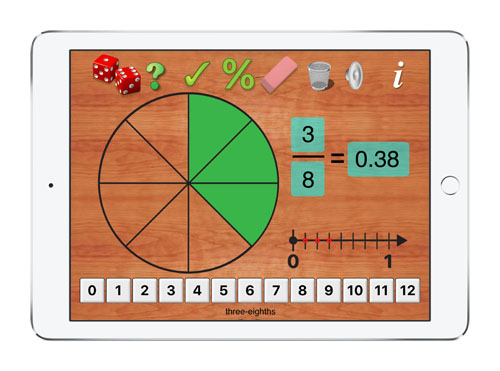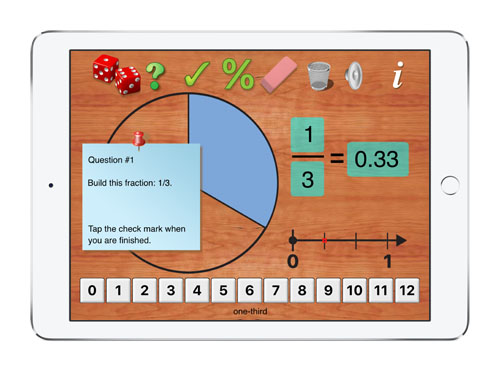Explore Concepts Related to Fractions with an Interactive Learning ToolFraction BuilderFraction Builder is an interactive tool for students to use to learn about fractions. Learn about naming fractions, equivalent fractions and comparing fractions. Fraction Builder is controlled by sliding tiles and tapping icons. As students build fractions, the fractions are shown as a numerator over a denominator, as a decimal, as parts of a whole and as a position on a number line. When the quiz icon is tapped questions automatically appear on a moveable note. Students slide tiles to answer the quiz question. Three different topics can be selected: 1. Naming Fractions 2. Equivalent Fractions 3. Comparing Fractions As the student uses the app, a scoreboard is keeps track of the student's progress and shows the number of problems attempted and also what percent of the problems were answer correctlyQ

Fraction Builder - A Powerful Teaching Tool

 \$1.99